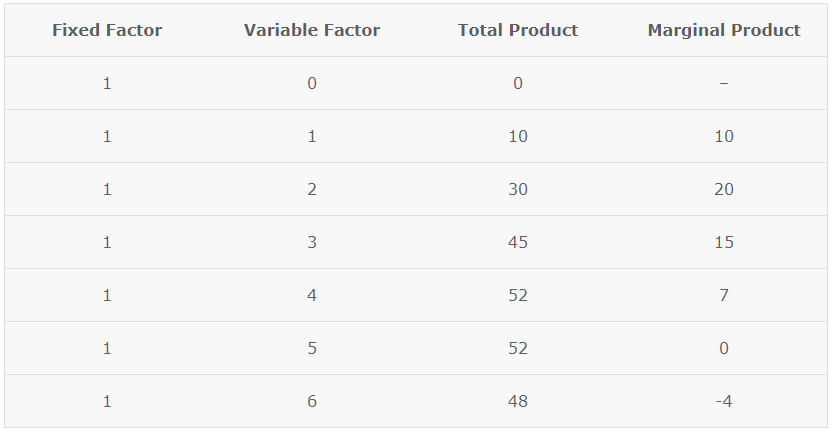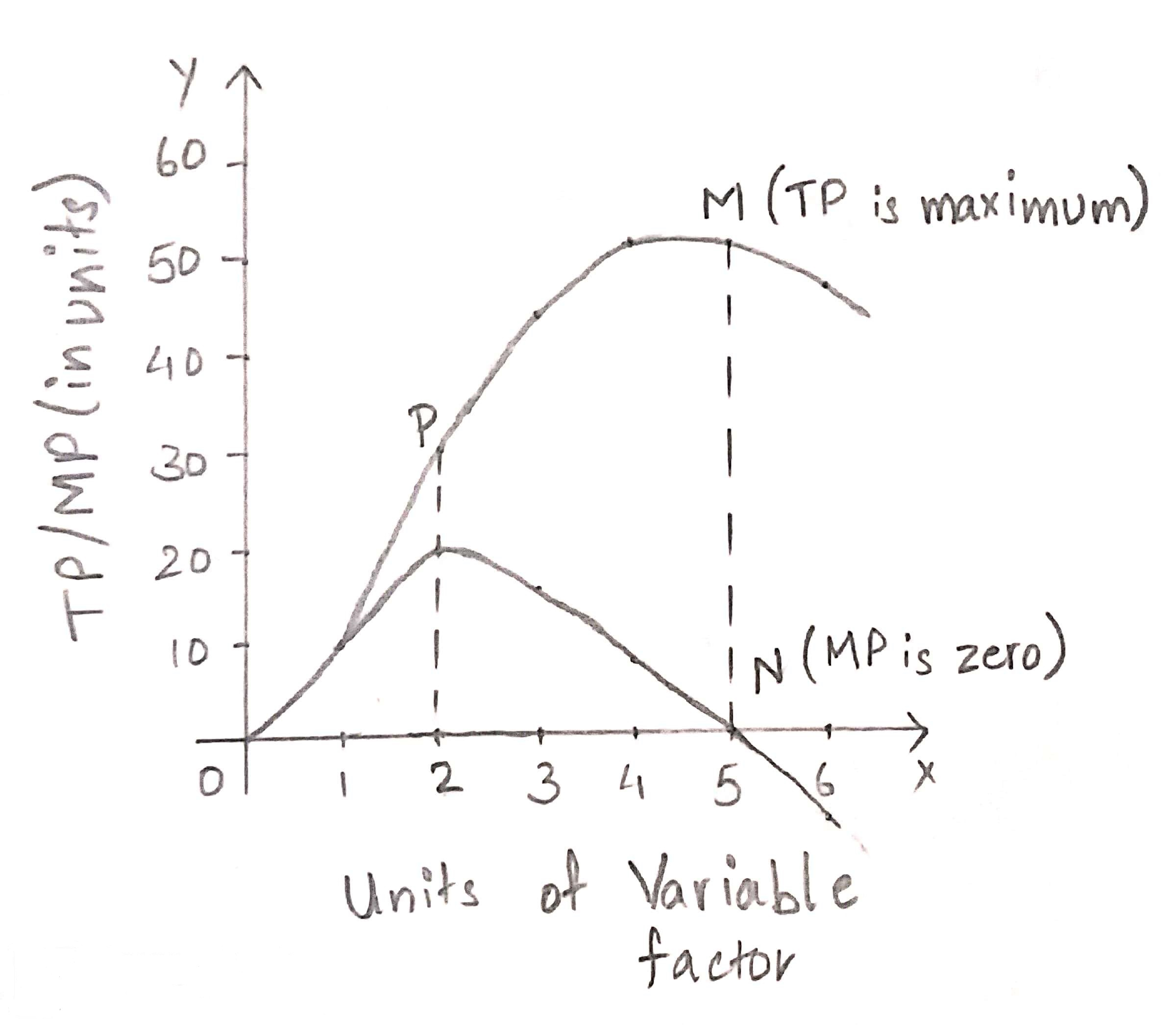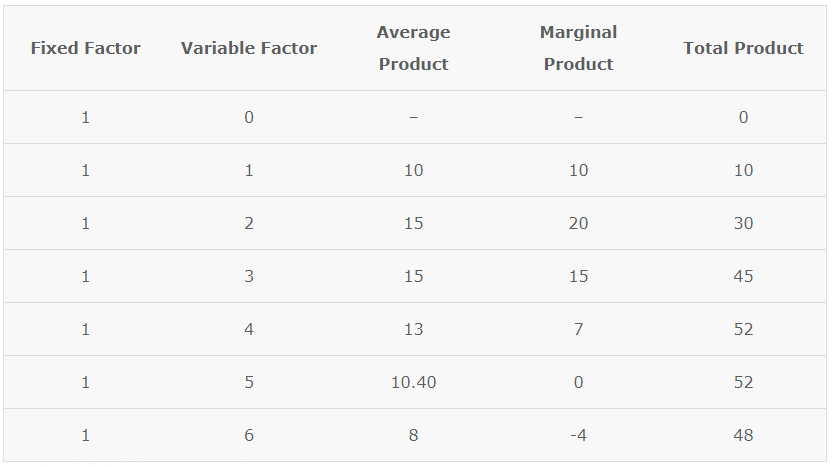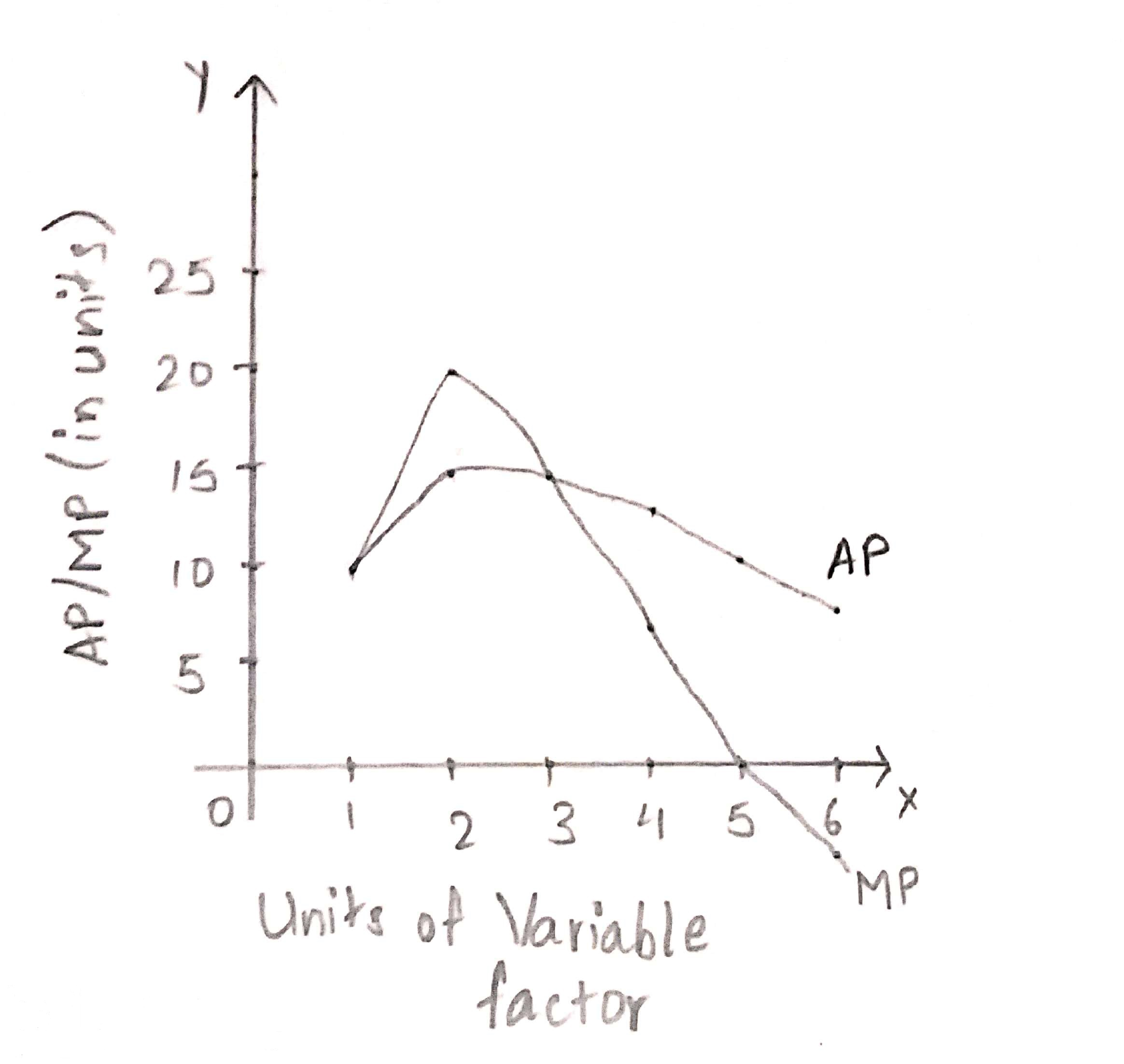# Relationship Between Total Product Average Product and Marginal Product

#### Topic Covered in this page

There is a unique relationship between total product (TP) and marginal product (MP) and between marginal product (MP) and average product (AP). It is very important to understand the relationship between these concepts in order to understand the process behind production by firms.

## Relationship between Total Product and Marginal Product

The relationship between TP and MP is explained through the Law of Variable Proportions. As long as the the TP increases at an increasing rate, the MP also increases. This goes on till MP reaches maximum. When TP increases at a diminishing rate, MP declines. This continues till the point where TP is at its highest. When TP reaches its maximum point, MP becomes zero. This concept can be better understood with the help of the following schedule and diagram.As you can see in the graph, TP increases at an increasing rate till point P, the point of inflextion, and till that point (i.e. the 2nd unit of variable factor), MP increases. Then, as the TP start increasing at a diminishing rate till point M when TP is maximum., the MP keeps declining and reaches zero at point N. This happens at the 6th unit of variable factor. After this point, the TP start decreasing and MP becomes negative, which can be seen when the 7th unit of variable factor is employed.

## Relationship between Marginal Product and Average Product

The marginal product (MP) and average product (AP) initially increase and then decrease due to the operation of the Law of Diminishing Marginal Returns. As long as MP is higher than AP, AP increases. At the highest point of AP, i.e. when AP is at its maximum, MP is equal to AP. When MP becomes lesser than AP, AP also starts to fall. Thereafter, both AP and MP fall, but MP becomes negative and AP remains positive. Also, MP falls at a faster rate as compared to AP. This can be better understood with the help of the following schedule and diagram.As seen in the above given graph, as long as MP is more than AP, AP rises, i.e. till the 2nd unit of variable factor. When MP and AP become equal at the 3rd unit, AP is at its highest. When MP becomes less than AP, from the 4th unit of variable factor, AP falls. After that, both MP and AP fall but the curve of MP is steeper than that of AP, and AP remains positive while MP becomes negative.